# PHYS 150 Physics I Prerequisite Corequisite Instructor title

• Slides: 77
Download presentation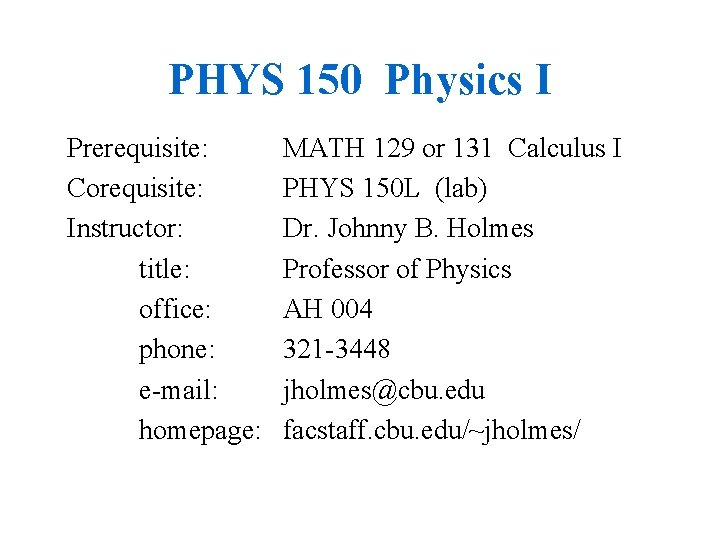PHYS 150 Physics I Prerequisite: Corequisite: Instructor: title: office: phone: e-mail: homepage: MATH 129 or 131 Calculus I PHYS 150 L (lab) Dr. Johnny B. Holmes Professor of Physics AH 004 321 -3448 [email protected] edu facstaff. cbu. edu/~jholmes/PHYS 150: Physics I A beginning course in physics covering the topics of kinematics, dynamics, gravitation, work, energy, momentum, rotational kinematics, and rotational dynamics. Warning: Some of these terms, especially the terms work, energy, and momentum, are used in everyday language but in physics they have very specific definitions. Prerequisites: algebra and basic trig, definition of derivative and integral, ability to differentiate and integrate power laws, sines & cosines, and exponentials. Corequisite: PHYS 150 L (the lab) PHYS 150 can substitute for PHYS 201 Introductory Physics I (note: you cannot get credit for both PHYS 150 and 201).PHYS 150: Physics I You all know some physics – that is, how the physical world works. This course is designed to challenge your “common sense”, and to suggest how to adjust your common sense when it is contrary to what actually happens. The course is also designed to expand your common sense. This course will also show you how to be quantitative about your predictions. This course will also try to give you some idea of how big or how small many things are.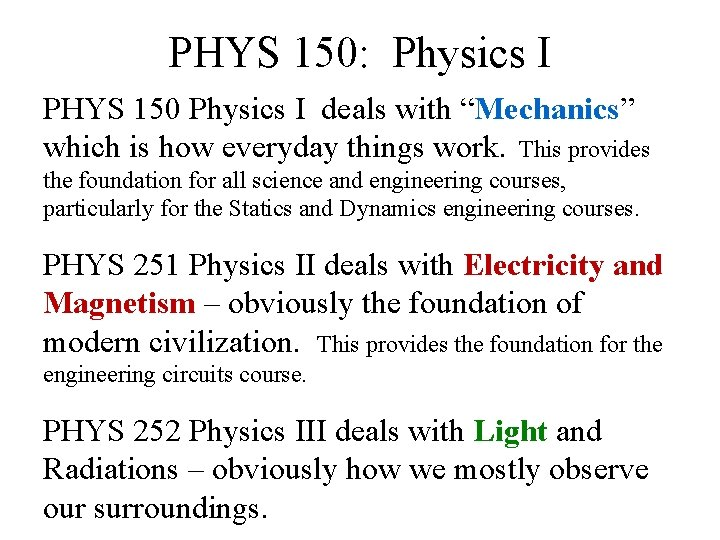PHYS 150: Physics I PHYS 150 Physics I deals with “Mechanics” which is how everyday things work. This provides the foundation for all science and engineering courses, particularly for the Statics and Dynamics engineering courses. PHYS 251 Physics II deals with Electricity and Magnetism – obviously the foundation of modern civilization. This provides the foundation for the engineering circuits course. PHYS 252 Physics III deals with Light and Radiations – obviously how we mostly observe our surroundings.Grading: (explained on syllabus) • 4 tests, each counts as one grade • 1 set of 9 regular collected homework problems which counts as one grade Note: You must get at least 65 on this grade to be eligible to pass the course • 2 sets of 10 computer homework programs where each set counts as one grade • final exam, which counts as 3 grades Total: 10 grades, final grade will be based on the average of these 10 grades. 100 – A – 93 – B – 82 – C – 70 – D – 65 – F - 0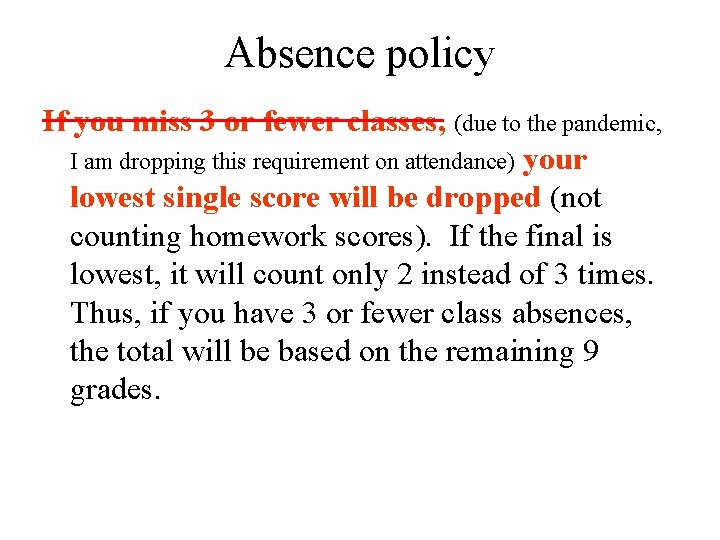Absence policy If you miss 3 or fewer classes, (due to the pandemic, I am dropping this requirement on attendance) your lowest single score will be dropped (not counting homework scores). If the final is lowest, it will count only 2 instead of 3 times. Thus, if you have 3 or fewer class absences, the total will be based on the remaining 9 grades.Regular Homework There are 9 regular collected homework problems for the semester. See the schedule on the syllabus. Due to the pandemic, you may submit them via e-mail with a word doc or picture of your work attached. These must be done and written up using the 7 step paradigm described in the syllabus and on the next slide. This paradigm is a waste of time for the problems with obvious solutions, but is good for attacking those problems that do not have obvious solutions. For these 9 problems, I want you to follow the 7 step paradigm even if the answer is obvious so you get practice using this paradigm. It is also a good way of communicating your thinking.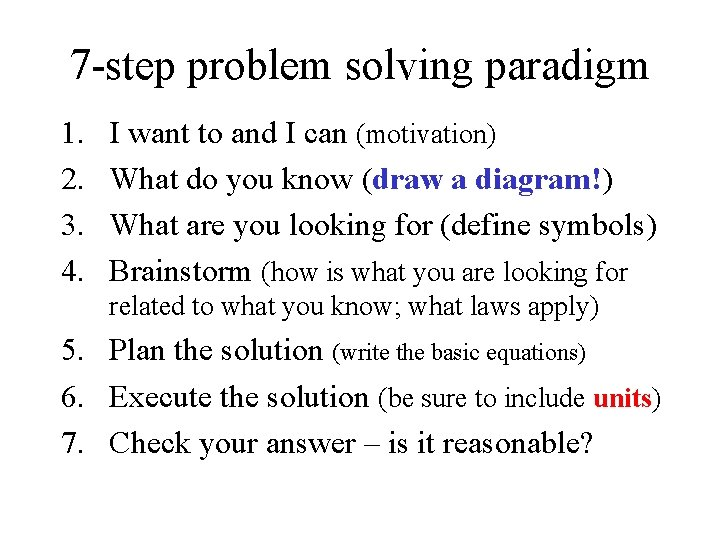7 -step problem solving paradigm 1. 2. 3. 4. I want to and I can (motivation) What do you know (draw a diagram!) What are you looking for (define symbols) Brainstorm (how is what you are looking for related to what you know; what laws apply) 5. Plan the solution (write the basic equations) 6. Execute the solution (be sure to include units) 7. Check your answer – is it reasonable?Computer Homework The 20 computer homework programs (each program consists of a problem set) are designed to give you graded practice. They emphasize getting the answer right the first time. The computer will tell you right away if your answer is correct or not, and if it is not correct it will often tell you how to get it right with numerical values so you can figure out if you missed the physics or had the physics right but messed up with the calculations. In most cases, you continue with the next problem, or start the program over if you want to get full credit.Computer Homework It is your task to actually get them correct. A random number generator will change the numbers so you will have to learn how to do them and not just remember the right answer. This “penalty” of starting over at the beginning, rather than with the problem you missed, to get full credit will cause you a lot of frustration, but the aim is to get you to think about your answers before you submit them. In practice, many problems have multiple steps, and you have to learn to double or triple check each step since only one wrong step will cause your final answer to be wrong. This “penalty” has the added benefit of getting you more practice with the early problems. To encourage you to get full credit, there is a bonus of 10 extra points if you get 100/100 (10/10 on 10 programs) on either or both of the computer homework grades.Tests The 4 tests and final exam emphasize familiarity, recognition and speed. The material on the tests should be somewhat familiar. You should be able to recognize the type of problem, identify the basic principles involved, and determine which techniques to apply. Partial credit for wrong or missing answers may be given if you show your work on the test paper.Study sheet This course emphasizes basic principles and problem solving, not memorization. To reduce the perceived need to memorize, you are permitted to bring to the tests one 8. 5” x 11” sheet of paper with information on one side. You may bring two study sheets to the final (writing on one side only, or one sheet with writing on both sides). Calculators are recommended, but calculators can not be shared, and no internet connected devices are permitted during tests. You should keep your hands, calculator, and eyes on the desk and not in your lap.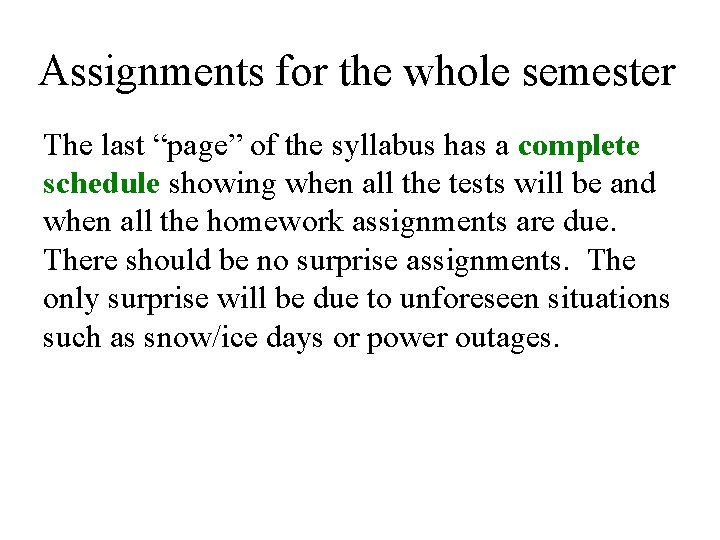Assignments for the whole semester The last “page” of the syllabus has a complete schedule showing when all the tests will be and when all the homework assignments are due. There should be no surprise assignments. The only surprise will be due to unforeseen situations such as snow/ice days or power outages.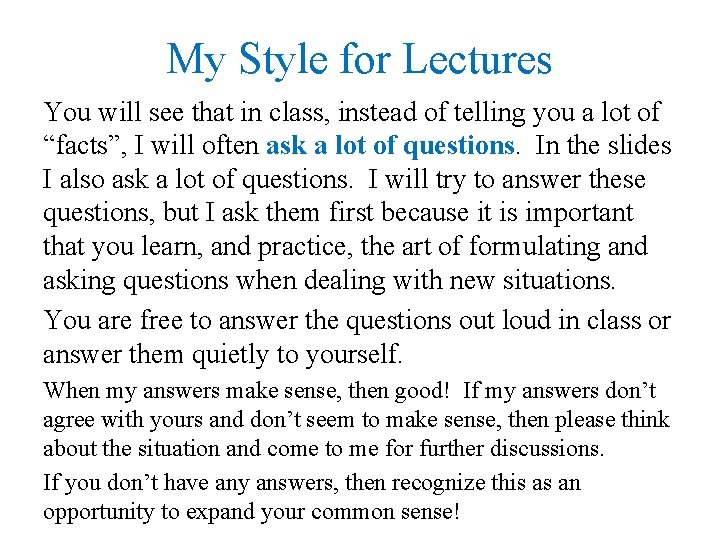My Style for Lectures You will see that in class, instead of telling you a lot of “facts”, I will often ask a lot of questions. In the slides I also ask a lot of questions. I will try to answer these questions, but I ask them first because it is important that you learn, and practice, the art of formulating and asking questions when dealing with new situations. You are free to answer the questions out loud in class or answer them quietly to yourself. When my answers make sense, then good! If my answers don’t agree with yours and don’t seem to make sense, then please think about the situation and come to me for further discussions. If you don’t have any answers, then recognize this as an opportunity to expand your common sense!OFFICE HOURS I would enjoy seeing you outside of class – whether to talk about homework, upcoming tests, or just talk about physics – whatever you are interested in. Office hours are linked from the course web page, but no formal appointment is necessary. So please feel that you are welcome to stop by my office anytime you see me in my office! In this semester of the pandemic, I suggest you use e -mail for your questions rather than face-to-face in my office. My office is right outside the classroom in AH 004. I’m usually in by 7 AM and before and after labs. By the way, I won’t think that you are “dumb” for doing this! On the contrary, it shows me you are interested in learning!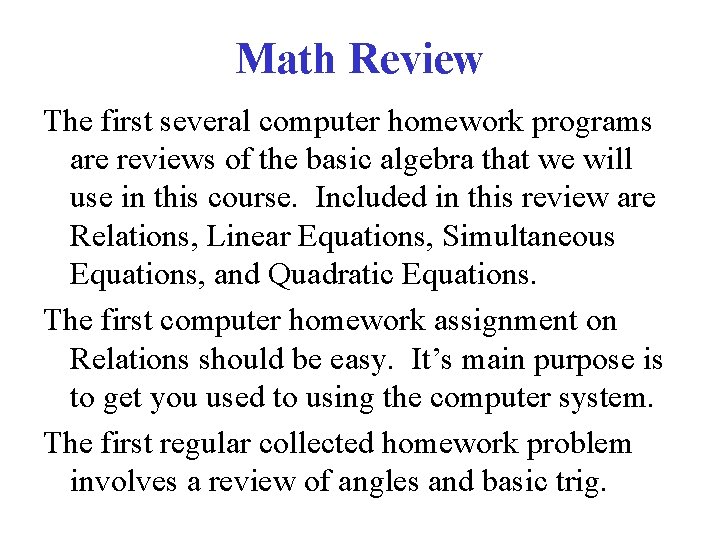Math Review The first several computer homework programs are reviews of the basic algebra that we will use in this course. Included in this review are Relations, Linear Equations, Simultaneous Equations, and Quadratic Equations. The first computer homework assignment on Relations should be easy. It’s main purpose is to get you used to using the computer system. The first regular collected homework problem involves a review of angles and basic trig.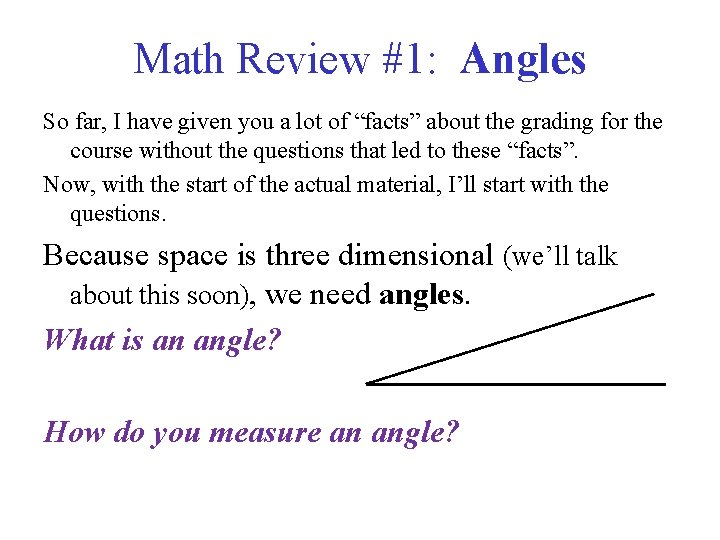Math Review #1: Angles So far, I have given you a lot of “facts” about the grading for the course without the questions that led to these “facts”. Now, with the start of the actual material, I’ll start with the questions. Because space is three dimensional (we’ll talk about this soon), we need angles. What is an angle? How do you measure an angle?Math Review #1: Angles How do you measure an angle? 1) in (fractions of) circles (cycles, rotations, and revolutions are different names for the same thing) 2) in degrees - but what is a degree? Why do they break the circle into 360 equal degrees? 3) in radians - but why use a weird number like 2 p for a circle? Why does p have the weird value of 3. 1415926535… ?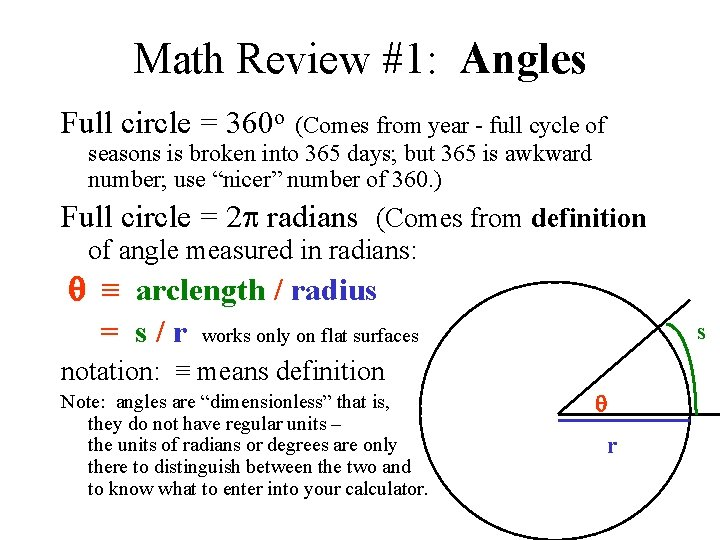Math Review #1: Angles Full circle = 360 o (Comes from year - full cycle of seasons is broken into 365 days; but 365 is awkward number; use “nicer” number of 360. ) Full circle = 2 p radians (Comes from definition of angle measured in radians: ≡ arclength / radius = s / r works only on flat surfaces s notation: ≡ means definition Note: angles are “dimensionless” that is, they do not have regular units – the units of radians or degrees are only there to distinguish between the two and to know what to enter into your calculator. r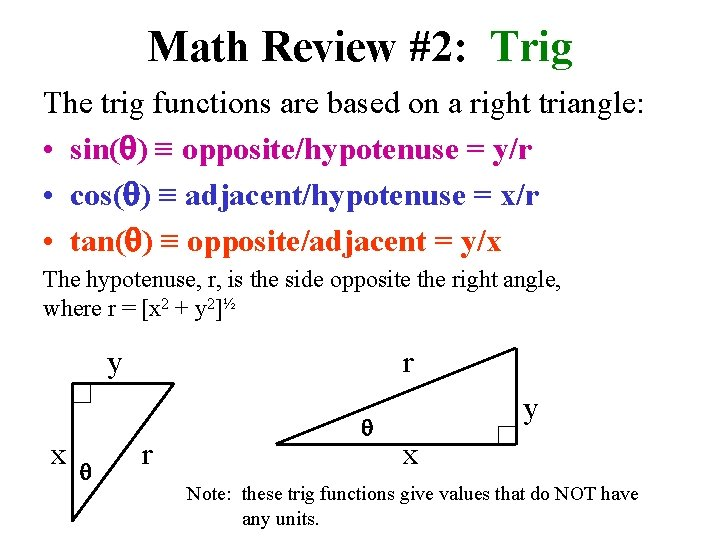Math Review #2: Trig The trig functions are based on a right triangle: • sin( ) ≡ opposite/hypotenuse = y/r • cos( ) ≡ adjacent/hypotenuse = x/r • tan( ) ≡ opposite/adjacent = y/x The hypotenuse, r, is the side opposite the right angle, where r = [x 2 + y 2]½ y x r r y x Note: these trig functions give values that do NOT have any units.Math Review #2: Trig • sin( ) ≡ opposite/hypotenuse = y/r • cos( ) ≡ adjacent/hypotenuse = x/r • tan( ) ≡ opposite/adjacent = y/x These functions are easy to see for angles between 0 o and 90 o. Do these trig functions work for angles less than 0 o and/or greater than 90 o? What are x, y, and r in these cases? > 90 oMath Review #2: Trig • sin( ) ≡ opposite/hypotenuse = y/r • cos( ) ≡ adjacent/hypotenuse = x/r • tan( ) ≡ opposite/adjacent = y/x Do these trig functions work for angles less than 0 o and/or greater than 90 o? Here we have y r y>0 and x<0 ; x we still have r = [x 2+y 2]½ > 0; and sin( ) = y/r > 0 , but cos( ) = x/r < 0. Check your calculator to see if you get reasonable answers for sine and cosine if is between 90 o and 180 o. What about angles in the third and fourth quadrants?Math Review #3: Linear Equations Review: One Linear Equation: ax + dy = c (we know a, d, and c; we don’t know x and y) Example: 5 x + 3 y = 35. Can you solve this equation?Math Review #3: Linear Equations Review: One Linear Equation: ax + dy = c example: 5 x + 3 y = 35 (we know a, d, and c; we don’t know x and y in the example, a = 5, d = 3, and c = 35) This is one equation in two unknowns. There are lots of correct answers to this. Several possible answers to the example equation are: (x=4, y=5), (x=7, y=0), (x=-5, y=20). Can you picture this (that is, draw a diagram)?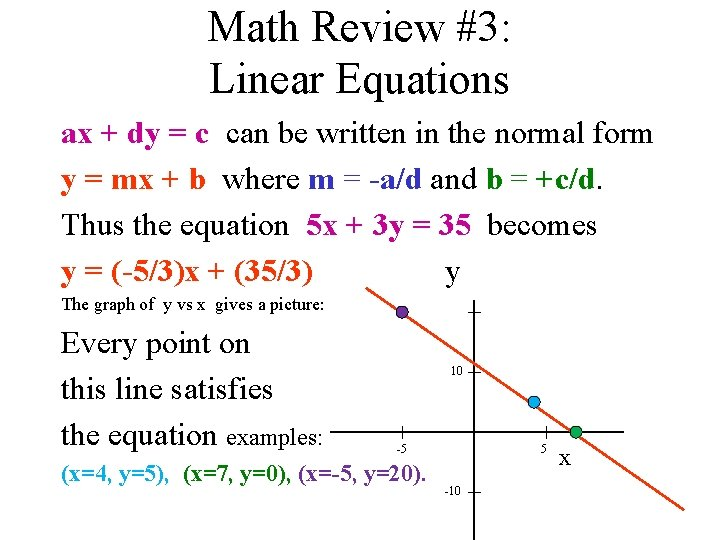Math Review #3: Linear Equations ax + dy = c can be written in the normal form y = mx + b where m = -a/d and b = +c/d. Thus the equation 5 x + 3 y = 35 becomes y = (-5/3)x + (35/3) y The graph of y vs x gives a picture: Every point on this line satisfies the equation examples: 10 -5 (x=4, y=5), (x=7, y=0), (x=-5, y=20). 5 -10 xMath Review #4: Simultaneous Equations However, if we have two equations with two unknowns, then there is usually just one possible answer. Can you picture this situation? In a later slide we will show a picture. Example: 5 x + 3 y = 35 AND 2 x - y = 3. In this case, we can solve for one unknown (say y) in terms of x: y = 2 x - 3 (using the second equation).Math Review #4: Simultaneous Equations 5 x + 3 y = 35 AND 2 x - y = 3. Using this relation for y: y = 2 x - 3 in the other (first) equation yields: 5 x + 3(2 x - 3) = 35 , or 5 x + 6 x - 9 = 35, or 11 x = 44, or x = 44/11 = 4 = x. Now we can use this value for x in the y = 2 x - 3 to get y = 2(4) - 3 = 5 = y.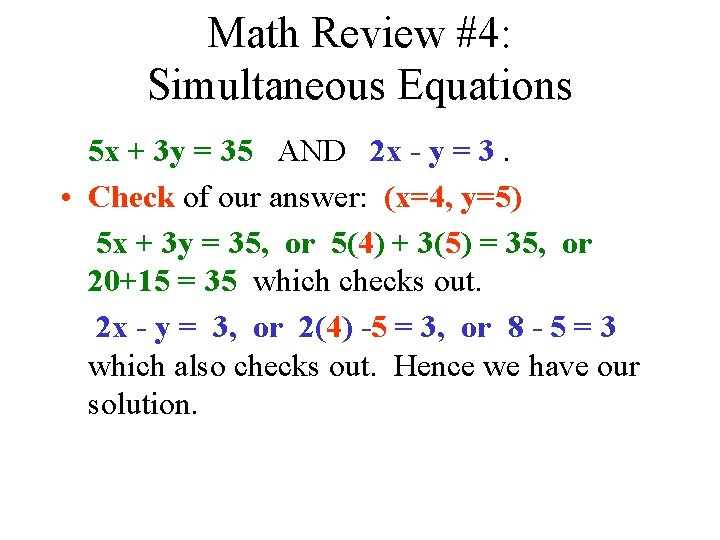Math Review #4: Simultaneous Equations 5 x + 3 y = 35 AND 2 x - y = 3. • Check of our answer: (x=4, y=5) 5 x + 3 y = 35, or 5(4) + 3(5) = 35, or 20+15 = 35 which checks out. 2 x - y = 3, or 2(4) -5 = 3, or 8 - 5 = 3 which also checks out. Hence we have our solution.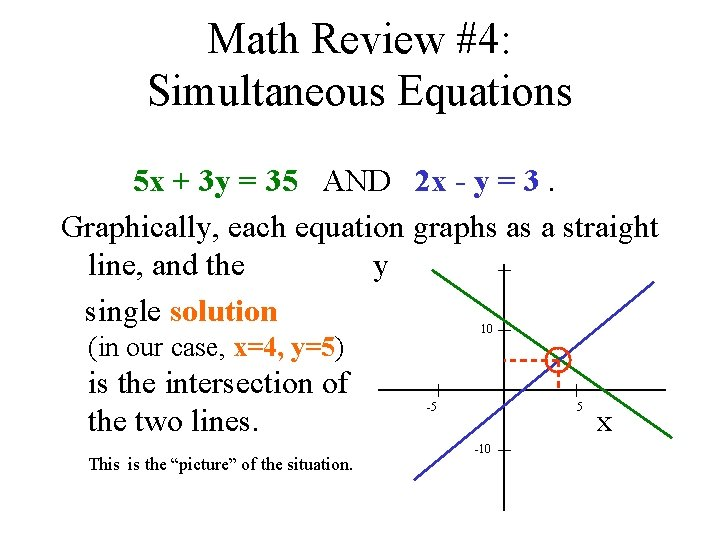Math Review #4: Simultaneous Equations 5 x + 3 y = 35 AND 2 x - y = 3. Graphically, each equation graphs as a straight line, and the y single solution 10 (in our case, x=4, y=5) is the intersection of the two lines. This is the “picture” of the situation. -5 5 -10 x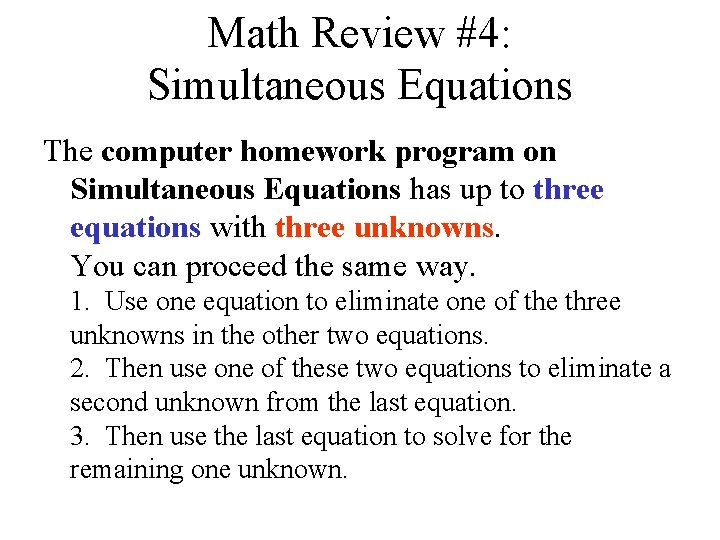Math Review #4: Simultaneous Equations The computer homework program on Simultaneous Equations has up to three equations with three unknowns. You can proceed the same way. 1. Use one equation to eliminate one of the three unknowns in the other two equations. 2. Then use one of these two equations to eliminate a second unknown from the last equation. 3. Then use the last equation to solve for the remaining one unknown.Math Review #4: Simultaneous Equations Can you picture 3 equations in 3 unknowns? 1. An equation like ax + by + cz = d can be pictured as a plane in 3 -D. 2. Two planes usually intersect giving a line. 3. That line then normally intersects with the third plane at one point. Exceptions: If two planes are parallel or if they are actually the same plane, they don’t intersect in a line. If a line is parallel to a plane, it doesn’t intersect the plane at a point.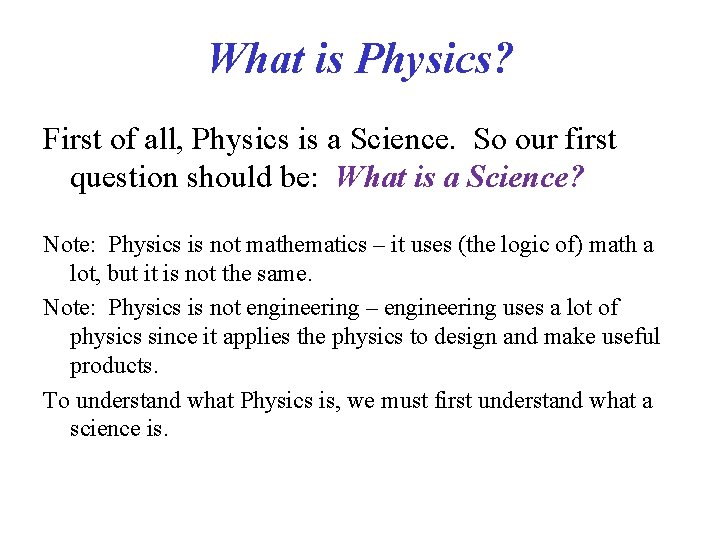What is Physics? First of all, Physics is a Science. So our first question should be: What is a Science? Note: Physics is not mathematics – it uses (the logic of) math a lot, but it is not the same. Note: Physics is not engineering – engineering uses a lot of physics since it applies the physics to design and make useful products. To understand what Physics is, we must first understand what a science is.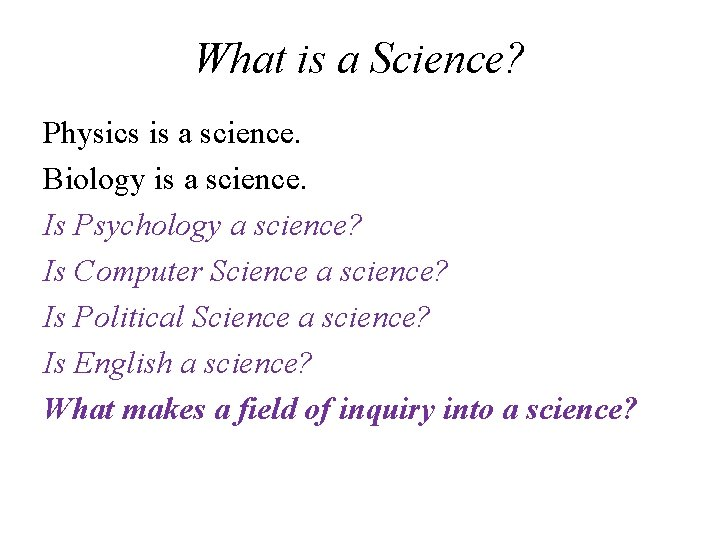What is a Science? Physics is a science. Biology is a science. Is Psychology a science? Is Computer Science a science? Is Political Science a science? Is English a science? What makes a field of inquiry into a science?Scientific Method What makes a field of inquiry into a science? Any field that employs the scientific method can be called a science. • So what is the Scientific Method? – What are the “steps” to this “method”? – Do you hypothesize (make a theory) or observe (gather data) first?Scientific Method 1. Define the “problem”: what are you studying? 2. Gather information (data). This should be repeatable (reproducible) by anyone else with the proper equipment. 3. Hypothesize (try to make “sense” of the data by trying to guess why it works or what law it seems to obey). This hypothesis should suggest how other things should work. So this leads to the need to: 4. TEST, but this is really gathering more information (really, back to step 2).Scientific Theories Two important things about scientific theories: 1. The data that theories are based on must be reproducible. 2. The theories must be testable – and hence falsifiable if they fail the test. There are theories about parallel universes and spatial dimensions more than the standard 3 (more on this later), but until they can be tested, they are more philosophical than scientific.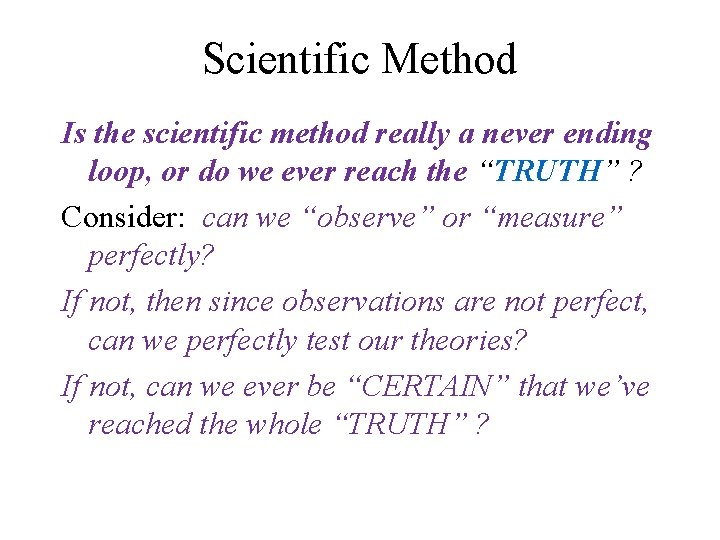Scientific Method Is the scientific method really a never ending loop, or do we ever reach the “TRUTH” ? Consider: can we “observe” or “measure” perfectly? If not, then since observations are not perfect, can we perfectly test our theories? If not, can we ever be “CERTAIN” that we’ve reached the whole “TRUTH” ?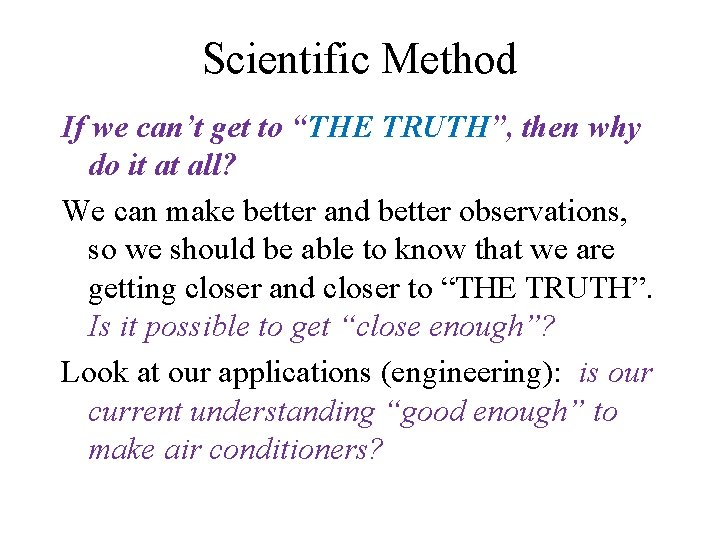Scientific Method If we can’t get to “THE TRUTH”, then why do it at all? We can make better and better observations, so we should be able to know that we are getting closer and closer to “THE TRUTH”. Is it possible to get “close enough”? Look at our applications (engineering): is our current understanding “good enough” to make air conditioners?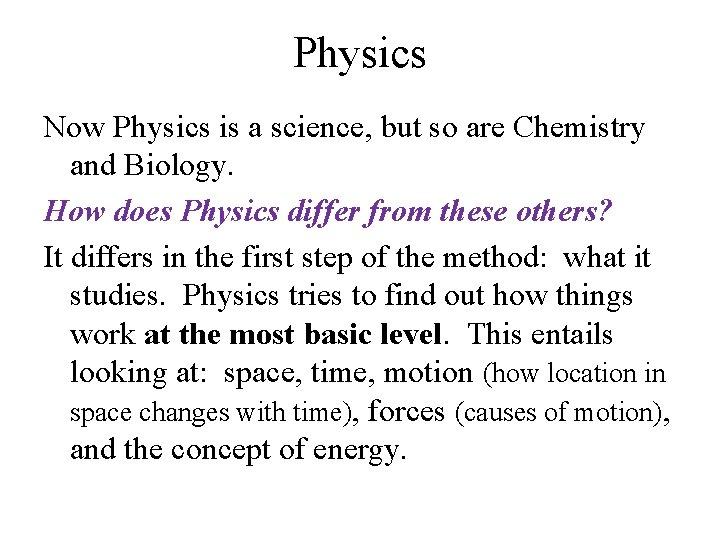Physics Now Physics is a science, but so are Chemistry and Biology. How does Physics differ from these others? It differs in the first step of the method: what it studies. Physics tries to find out how things work at the most basic level. This entails looking at: space, time, motion (how location in space changes with time), forces (causes of motion), and the concept of energy.Metric System Since physics is a science, and science deals with observations, physics deals with MEASUREMENTS. What and how do we measure? What do we use as standards for our measurements? In this course we will look at common units of measurement as well as the METRIC units. CAUTION: Almost all answers in physics will have UNITS – unlike answers in math courses. You will lose points if you forget to include units with your answers. Angles and trig functions (such as sine and cosine functions) do not have units, but be sure to use the correct degrees or radians when you calculate trig function values.Metric System: Basic quantities Some measurements are basic, i. e. , they can’t be defined in terms of other things, and some are combinations of other more basic ones: Here are the basics: (MKS system) length (in Meters) amount of “stuff” called mass (in Kilograms) time (in Seconds) In contrast, here are some of the combinations: speed (distance per time: m/s, mph) area (distance times another distance: m 2, ft 2) lots of other thingsPrefixes The basic metric unit for length is the meter. We can indicate a multiple of meters or a fraction of a meter by using prefixes: • centimeter (cm) =. 01 meters = 10 -2 m • millimeter (mm) =. 001 meters = 10 -3 m • micrometer ( m) =. 000001 meters = 10 -6 m • nanometer (nm) =. 00001 meters = 10 -9 m • picometer (pm) =. 0000001 meters = 10 -12 m (these prefixes can be used with any unit, not just with meters)Prefixes – cont. (The prefixes on this page will not be used in this course, but you may run into them in future courses. ) • • femto: atto: zepto yocto (fg) = 10 -15 (ag) = 10 -18 (zg) = 10 -21 (yg) = 10 -24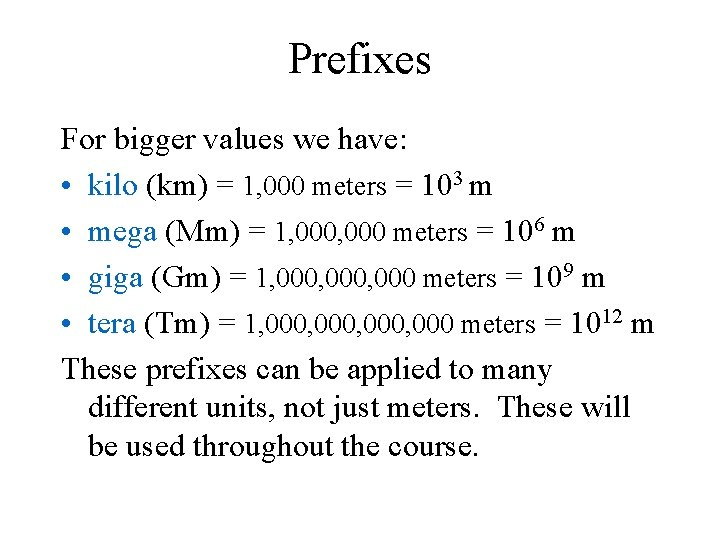Prefixes For bigger values we have: • kilo (km) = 1, 000 meters = 103 m • mega (Mm) = 1, 000 meters = 106 m • giga (Gm) = 1, 000, 000 meters = 109 m • tera (Tm) = 1, 000, 000 meters = 1012 m These prefixes can be applied to many different units, not just meters. These will be used throughout the course.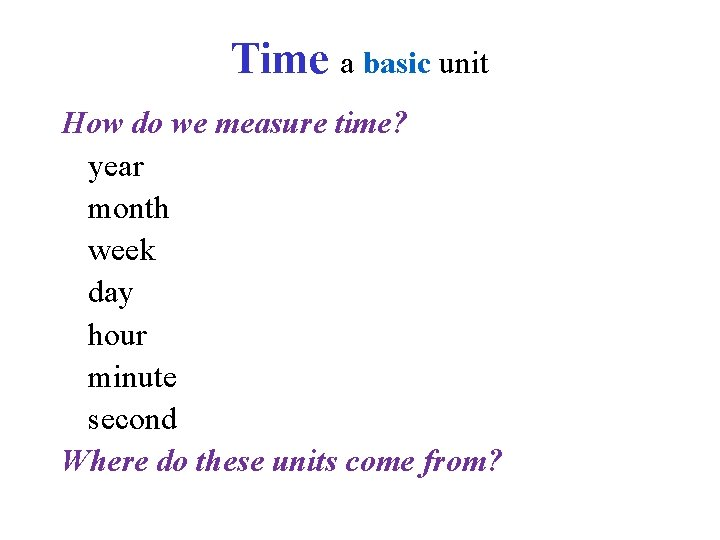Time a basic unit How do we measure time? year month week day hour minute second Where do these units come from?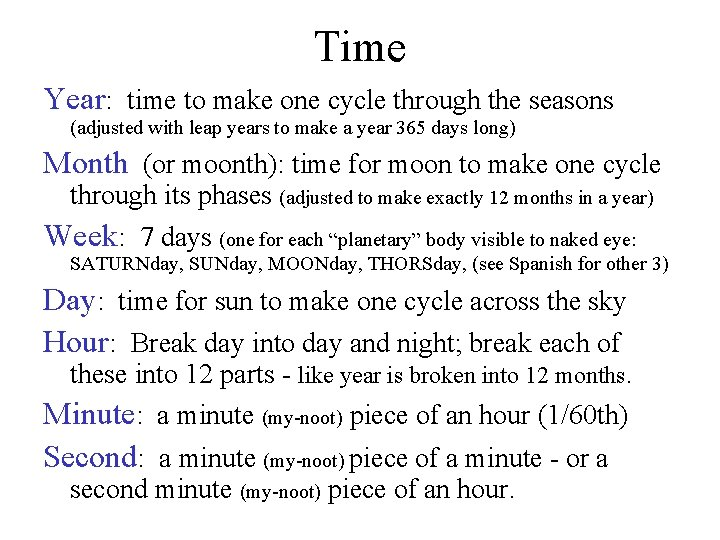Time Year: time to make one cycle through the seasons (adjusted with leap years to make a year 365 days long) Month (or moonth): time for moon to make one cycle through its phases (adjusted to make exactly 12 months in a year) Week: 7 days (one for each “planetary” body visible to naked eye: SATURNday, SUNday, MOONday, THORSday, (see Spanish for other 3) Day: time for sun to make one cycle across the sky Hour: Break day into day and night; break each of these into 12 parts - like year is broken into 12 months. Minute: a minute (my-noot) piece of an hour (1/60 th) Second: a minute (my-noot) piece of a minute - or a second minute (my-noot) piece of an hour.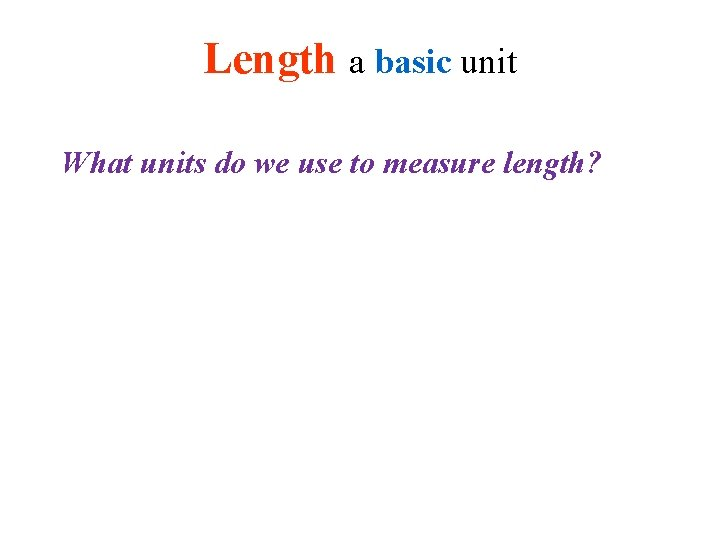Length a basic unit What units do we use to measure length?Length Foot (whose foot? ) Inch (length of a section of your finger) Mile (1, 000 double paces of a Roman legion) Nautical Mile (1 arc minute of angle around the earth = 1. 15 miles) [an arc min = 1/60 of a degree; circumference of earth ~ 25, 000 miles, so 25, 000 miles/360 o = 69 miles/1 o * 1 o/60 arc min = 1. 15 miles/arc min] Shortest distance is not the straight line on a flat map, but rather the distance along a great circle – one with a center of the circle at the earth’s center. League (3 miles), fathom (6 feet) , chain (100 links either 20 yards or 100 feet, or 10 yards in football) , etc. Meter The meter was originally defined as one ten-millionth (0. 0000001 or 10 -7) of the distance from the geographic north pole to the equator as measured over the earth's surface in a great circle passing through Paris, France, . One meter is now defined as the distance traveled by a ray of electromagnetic (EM) energy through a vacuum in 1/299, 792, 458 (3. 33564095 x 10 -9) of a second. (We’ll worry about MASS, a basic unit, later. )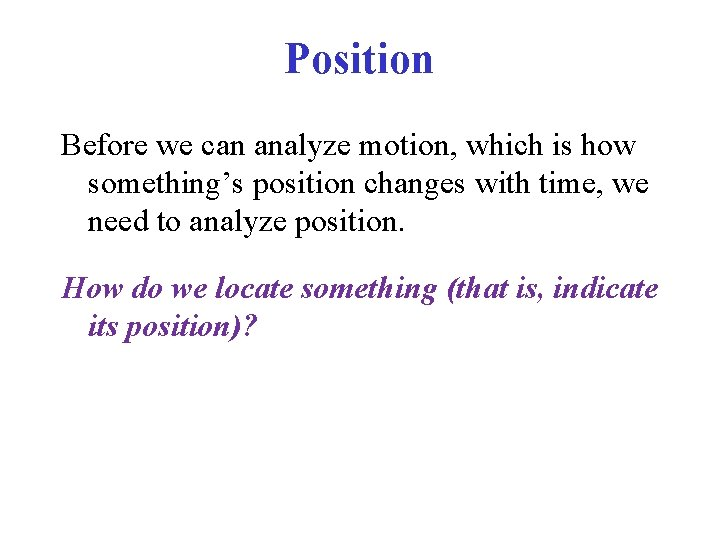Position Before we can analyze motion, which is how something’s position changes with time, we need to analyze position. How do we locate something (that is, indicate its position)?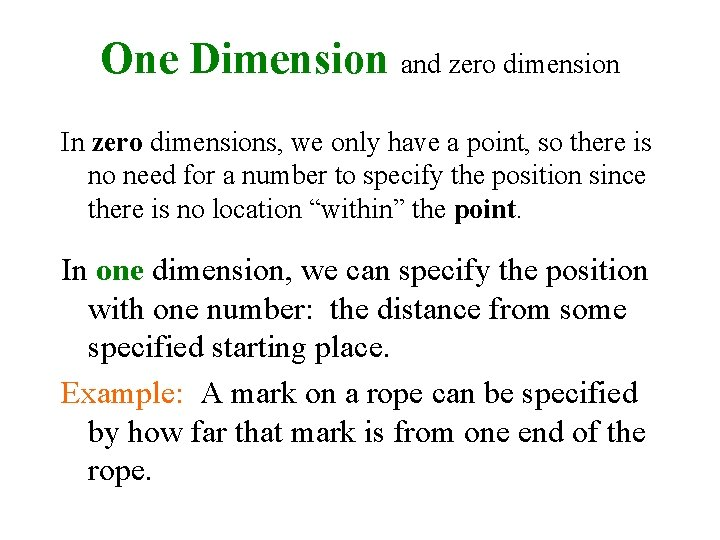One Dimension and zero dimension In zero dimensions, we only have a point, so there is no need for a number to specify the position since there is no location “within” the point. In one dimension, we can specify the position with one number: the distance from some specified starting place. Example: A mark on a rope can be specified by how far that mark is from one end of the rope.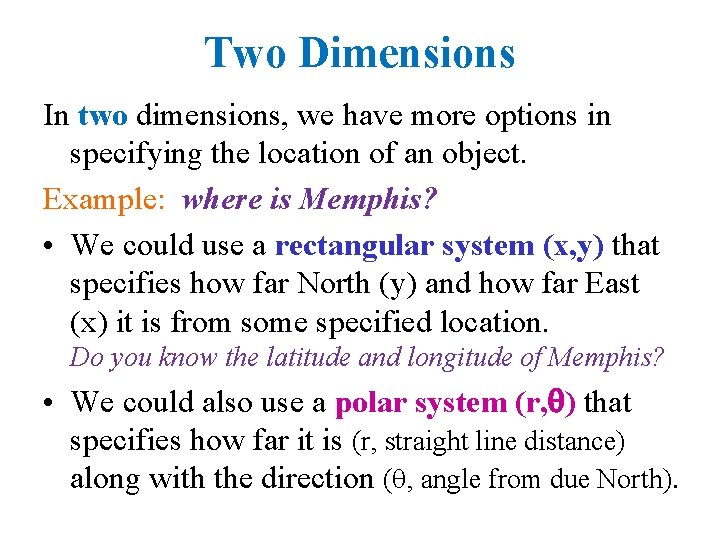Two Dimensions In two dimensions, we have more options in specifying the location of an object. Example: where is Memphis? • We could use a rectangular system (x, y) that specifies how far North (y) and how far East (x) it is from some specified location. Do you know the latitude and longitude of Memphis? • We could also use a polar system (r, ) that specifies how far it is (r, straight line distance) along with the direction ( , angle from due North).Three Dimensions Notice that both systems need TWO numbers - hence the TWO DIMENSIONS. With THREE dimensions, we need three numbers and we have even more options. Example: Locate an airplane in the sky. Some of the options are: rectangular (x, y, z), spherical (r, , ), cylindrical (r, , z). Could you make up a fourth system to determine the position inside a doughnut?Number of spatial dimensions • It is easy to see the need for three dimensions. Do we need FOUR? Sometimes time is counted as a fourth dimension, but it is a temporal dimension, not a spatial dimension. • What would a fourth dimension be like? • Experimentally, how many spatial dimensions do we have? • Most of the time in this course we will work in two dimensions since many cases can be reduced to this and it is mathematically easier. • More questions: Is space “flat”? Is the earth’s surface “flat”? Open versus Closed Universe? Normal Euclidian geometry is based on space being flat with parallel lines never meeting or diverging. The surface of the earth is an example of a non-Euclidian surface: if you are in Los Angeles and follow a longitudinal line going due North, you will meet another person from New York who follows another longitudinal line that is also going due North – at the North Pole. Euclidian geometry works well on the Earth as long as we have small distances, but gets worse on the spherical Earth as the distances get bigger. We’ll confine ourselves to Euclidian geometry in this course.Vectors How do we work with a quantity that needs two (or more) numbers to specify it (like position does)? We can work either with a group of numbers sometimes put in parenthesis, or we can work with unit vectors that we add together. For the position vector: (x, y), or x*x + y*y (where x called a unit vector indicates the x direction and x indicates how far in that direction), or x*i + y*j (where i is the unit vector in the x direction and j is the unit vector in the y direction). Both of the above are in rectangular form.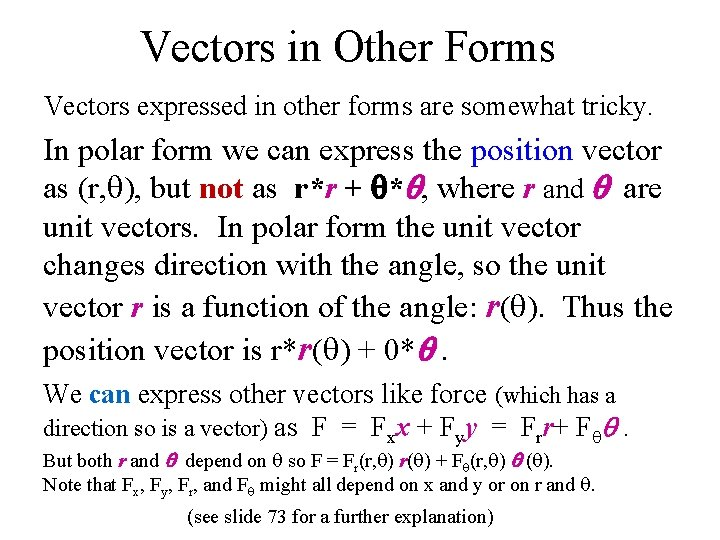Vectors in Other Forms Vectors expressed in other forms are somewhat tricky. In polar form we can express the position vector as (r, ), but not as r*r + * , where r and are unit vectors. In polar form the unit vector changes direction with the angle, so the unit vector r is a function of the angle: r( ). Thus the position vector is r*r( ) + 0* . We can express other vectors like force (which has a direction so is a vector) as F = Fxx + Fyy = Frr+ F . But both r and depend on so F = Fr(r, ) r( ) + F (r, ) ( ). Note that Fx, Fy, Fr, and F might all depend on x and y or on r and . (see slide 73 for a further explanation)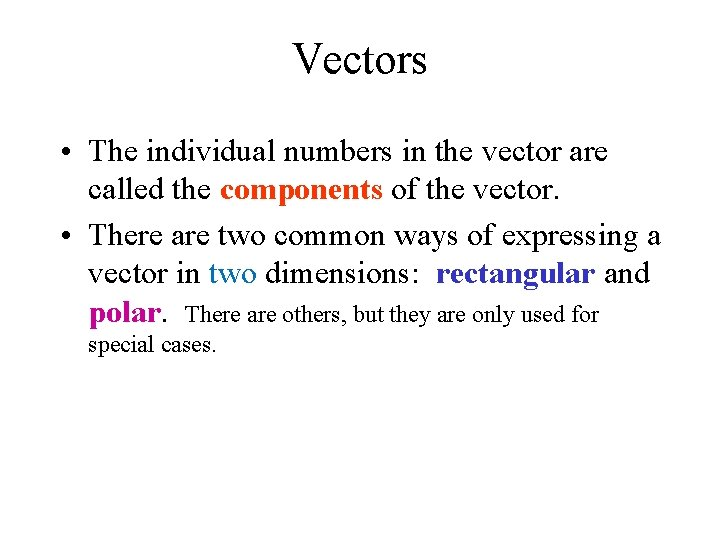Vectors • The individual numbers in the vector are called the components of the vector. • There are two common ways of expressing a vector in two dimensions: rectangular and polar. There are others, but they are only used for special cases.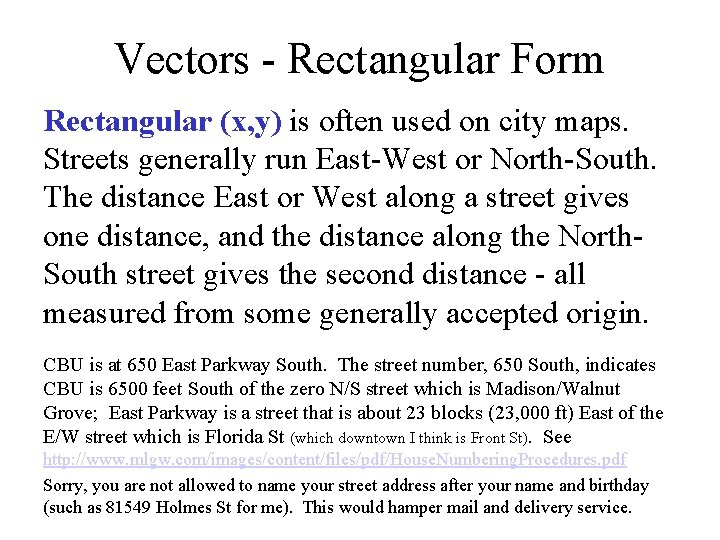Vectors - Rectangular Form Rectangular (x, y) is often used on city maps. Streets generally run East-West or North-South. The distance East or West along a street gives one distance, and the distance along the North. South street gives the second distance - all measured from some generally accepted origin. CBU is at 650 East Parkway South. The street number, 650 South, indicates CBU is 6500 feet South of the zero N/S street which is Madison/Walnut Grove; East Parkway is a street that is about 23 blocks (23, 000 ft) East of the E/W street which is Florida St (which downtown I think is Front St). See http: //www. mlgw. com/images/content/files/pdf/House. Numbering. Procedures. pdf Sorry, you are not allowed to name your street address after your name and birthday (such as 81549 Holmes St for me). This would hamper mail and delivery service.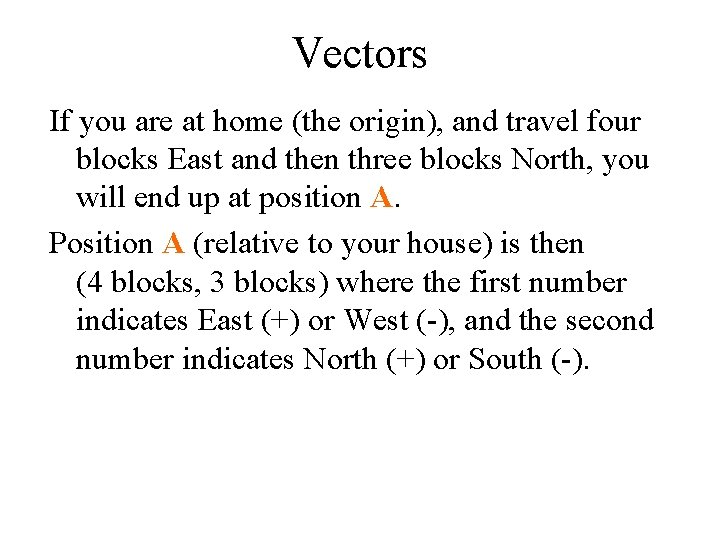Vectors If you are at home (the origin), and travel four blocks East and then three blocks North, you will end up at position A. Position A (relative to your house) is then (4 blocks, 3 blocks) where the first number indicates East (+) or West (-), and the second number indicates North (+) or South (-).Vectors If you had a helicopter or could walk directly there, it would be shorter to actually head straight there. A How do you specify 3 bl the location of point A this way? 4 blVectors - Polar Form • The distance can be calculated by the Pythagorian Theorem: r = [ x 2 + y 2 ] = [ (4 bl)2 + (3 bl)2 ] = 5 bl. A • The angle can be calculated 5 bl 3 bl using the tangent function: = tan-1 (y/x) = tan-1 (3 bl / 4 bl) = 37 o 4 bl Notice that this angle is measured counterclockwise from due East, not the compass angle which would be measured clockwise from due North.Transformation Equations These two equations are called the rectangular to polar transformation equations: r = [ x 2 + y 2 ] = tan-1(y/x). (that is, knowing x and y you can calculate r and θ) Do these work for all values of x and y, including negative values?Transformation Equations r = [ x 2 + y 2 ] The r equation does work all the time since when you square a positive or negative value, you still end up with a positive value. Thus r will always be positive (or zero), never negative.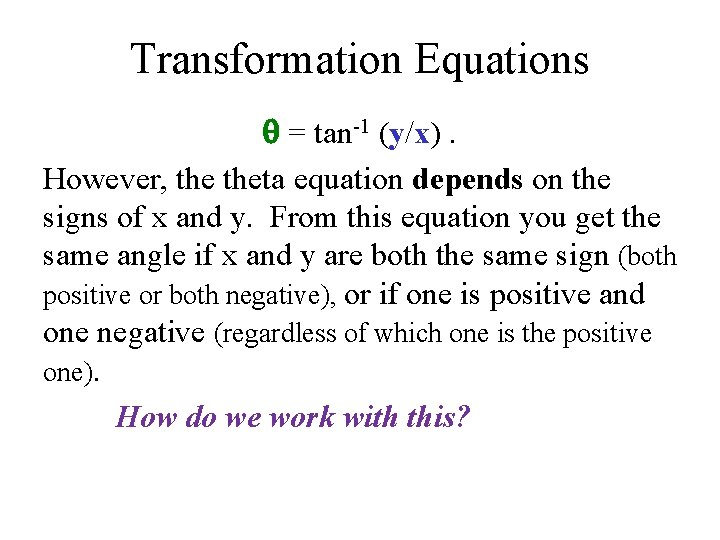Transformation Equations = tan-1 (y/x). However, theta equation depends on the signs of x and y. From this equation you get the same angle if x and y are both the same sign (both positive or both negative), or if one is positive and one negative (regardless of which one is the positive one). How do we work with this?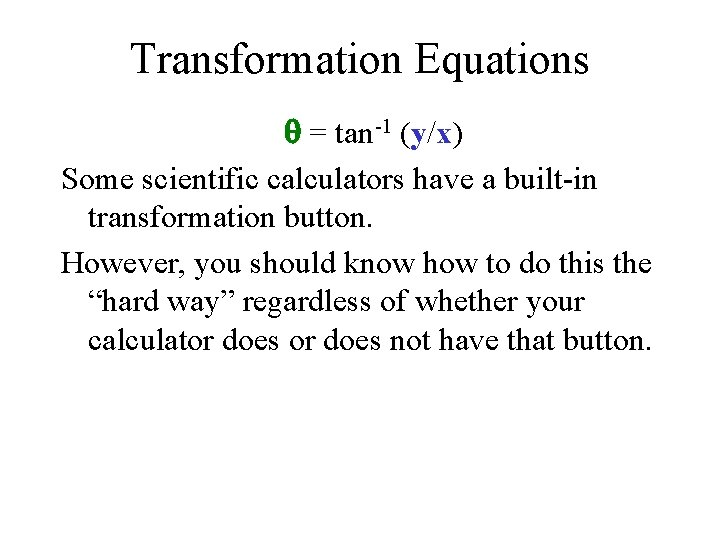Transformation Equations = tan-1 (y/x) Some scientific calculators have a built-in transformation button. However, you should know how to do this the “hard way” regardless of whether your calculator does not have that button.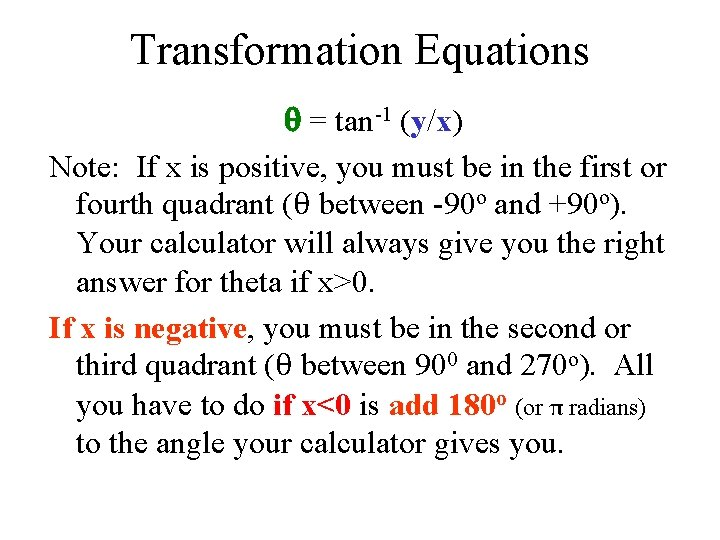Transformation Equations = tan-1 (y/x) Note: If x is positive, you must be in the first or fourth quadrant (θ between -90 o and +90 o). Your calculator will always give you the right answer for theta if x>0. If x is negative, you must be in the second or third quadrant (θ between 900 and 270 o). All you have to do if x<0 is add 180 o (or π radians) to the angle your calculator gives you.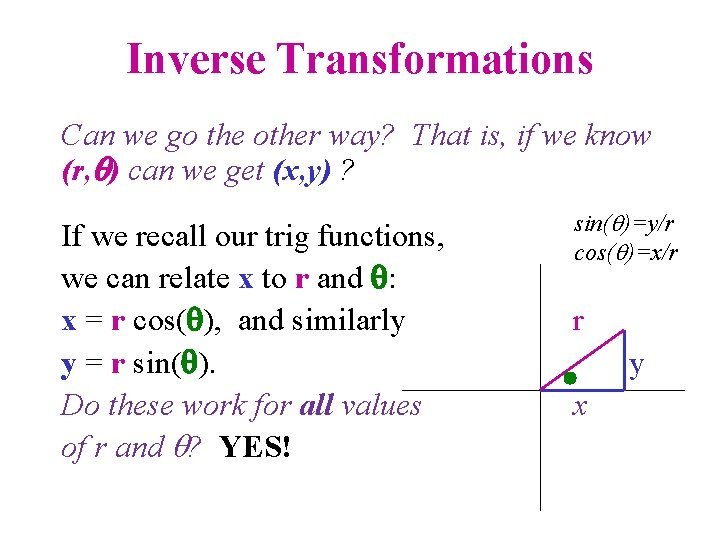Inverse Transformations Can we go the other way? That is, if we know (r, ) can we get (x, y) ? If we recall our trig functions, we can relate x to r and : x = r cos( ), and similarly y = r sin( ). Do these work for all values of r and ? YES! sin( )=y/r cos( )=x/r r y x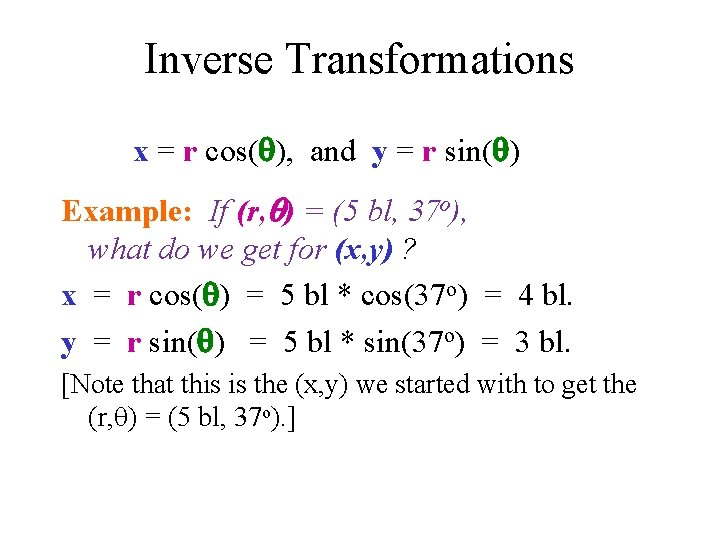Inverse Transformations x = r cos( ), and y = r sin( ) Example: If (r, ) = (5 bl, 37 o), what do we get for (x, y) ? x = r cos( ) = 5 bl * cos(37 o) = 4 bl. y = r sin( ) = 5 bl * sin(37 o) = 3 bl. [Note that this is the (x, y) we started with to get the (r, ) = (5 bl, 37 o). ]Motion involves changing the position of an object during a time interval. If position is a vector, then the change in position should also be a vector. The change in position involves the difference between the final and initial positions. But before we concern ourselves with subtraction (finding a difference), we need to look at addition!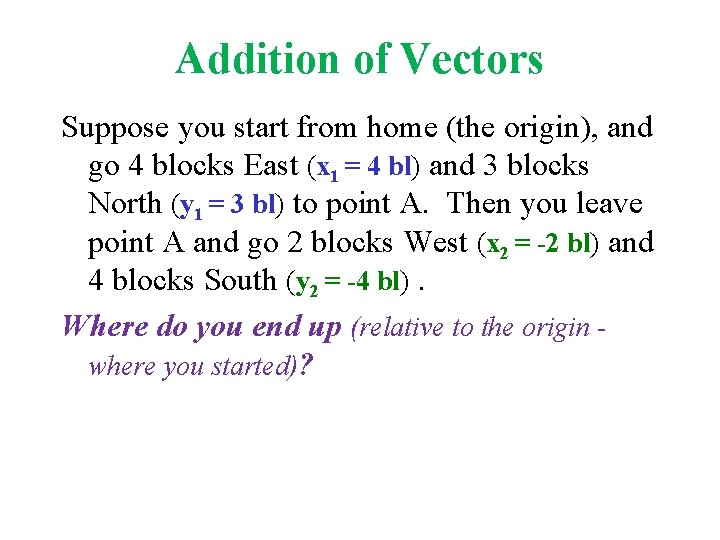Addition of Vectors Suppose you start from home (the origin), and go 4 blocks East (x 1 = 4 bl) and 3 blocks North (y 1 = 3 bl) to point A. Then you leave point A and go 2 blocks West (x 2 = -2 bl) and 4 blocks South (y 2 = -4 bl). Where do you end up (relative to the origin where you started)?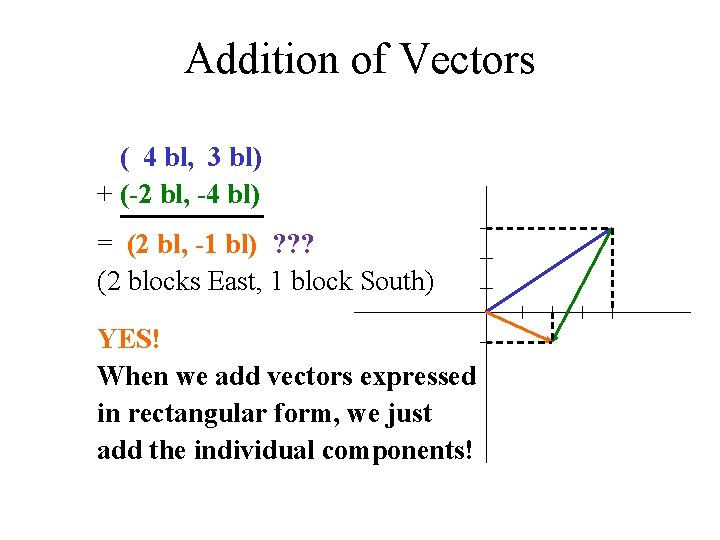Addition of Vectors ( 4 bl, 3 bl) + (-2 bl, -4 bl) = (2 bl, -1 bl) ? ? ? (2 blocks East, 1 block South) YES! When we add vectors expressed in rectangular form, we just add the individual components!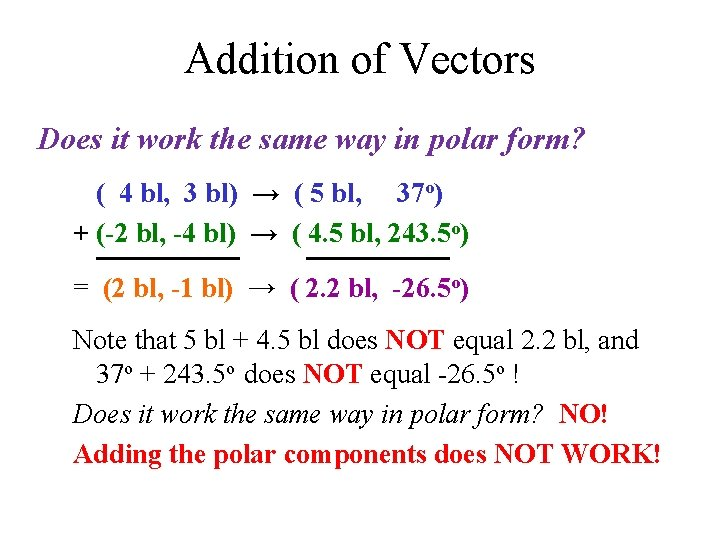Addition of Vectors Does it work the same way in polar form? ( 4 bl, 3 bl) → ( 5 bl, 37 o) + (-2 bl, -4 bl) → ( 4. 5 bl, 243. 5 o) = (2 bl, -1 bl) → ( 2. 2 bl, -26. 5 o) Note that 5 bl + 4. 5 bl does NOT equal 2. 2 bl, and 37 o + 243. 5 o does NOT equal -26. 5 o ! Does it work the same way in polar form? NO! Adding the polar components does NOT WORK!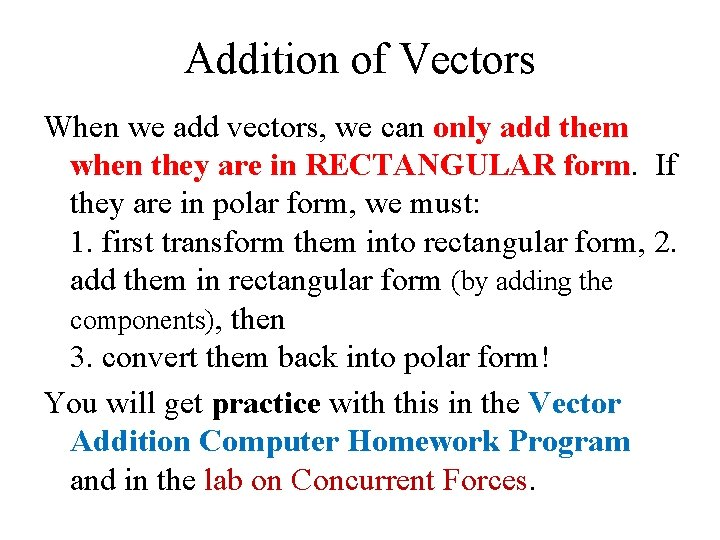Addition of Vectors When we add vectors, we can only add them when they are in RECTANGULAR form. If they are in polar form, we must: 1. first transform them into rectangular form, 2. add them in rectangular form (by adding the components), then 3. convert them back into polar form! You will get practice with this in the Vector Addition Computer Homework Program and in the lab on Concurrent Forces.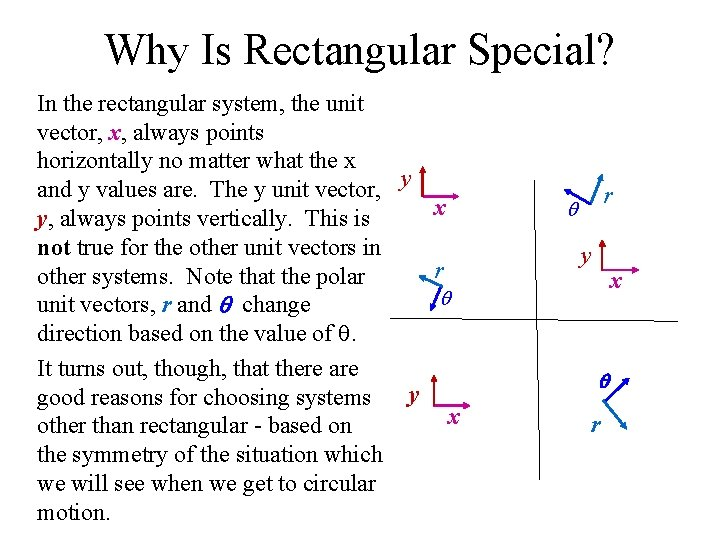Why Is Rectangular Special? In the rectangular system, the unit vector, x, always points horizontally no matter what the x and y values are. The y unit vector, y x y, always points vertically. This is not true for the other unit vectors in r other systems. Note that the polar unit vectors, r and change direction based on the value of . It turns out, though, that there are y good reasons for choosing systems x other than rectangular - based on the symmetry of the situation which we will see when we get to circular motion. r y x r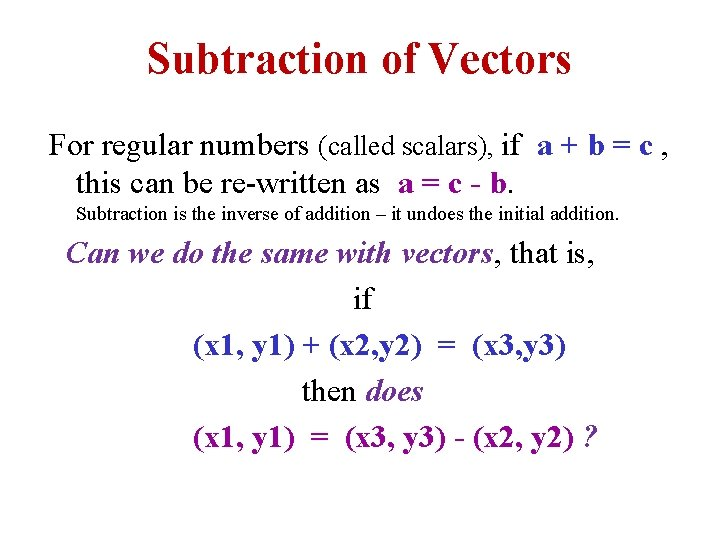Subtraction of Vectors For regular numbers (called scalars), if a + b = c , this can be re-written as a = c - b. Subtraction is the inverse of addition – it undoes the initial addition. Can we do the same with vectors, that is, if (x 1, y 1) + (x 2, y 2) = (x 3, y 3) then does (x 1, y 1) = (x 3, y 3) - (x 2, y 2) ?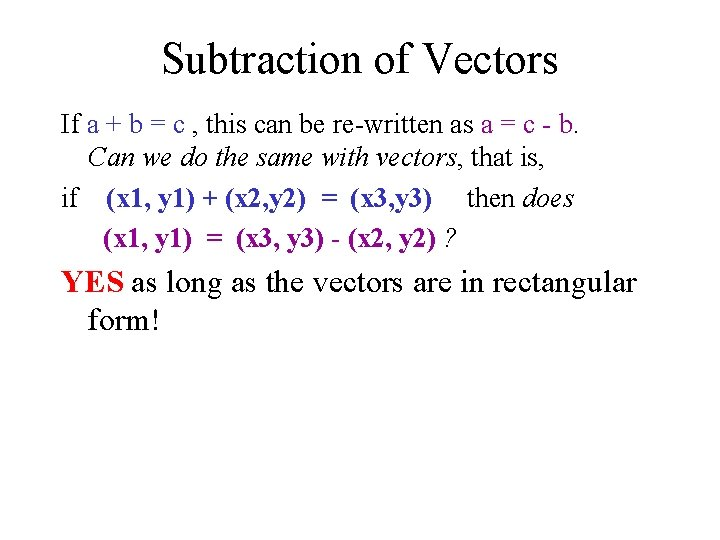Subtraction of Vectors If a + b = c , this can be re-written as a = c - b. Can we do the same with vectors, that is, if (x 1, y 1) + (x 2, y 2) = (x 3, y 3) then does (x 1, y 1) = (x 3, y 3) - (x 2, y 2) ? YES as long as the vectors are in rectangular form!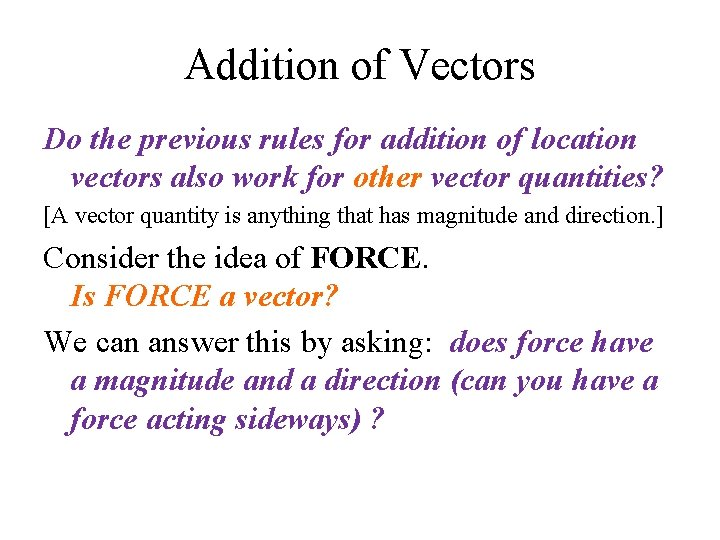Addition of Vectors Do the previous rules for addition of location vectors also work for other vector quantities? [A vector quantity is anything that has magnitude and direction. ] Consider the idea of FORCE. Is FORCE a vector? We can answer this by asking: does force have a magnitude and a direction (can you have a force acting sideways) ?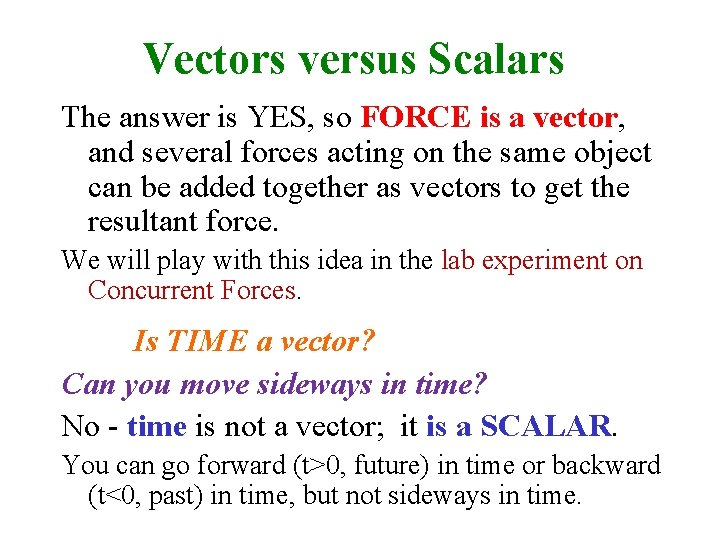Vectors versus Scalars The answer is YES, so FORCE is a vector, and several forces acting on the same object can be added together as vectors to get the resultant force. We will play with this idea in the lab experiment on Concurrent Forces. Is TIME a vector? Can you move sideways in time? No - time is not a vector; it is a SCALAR. You can go forward (t>0, future) in time or backward (t<0, past) in time, but not sideways in time.